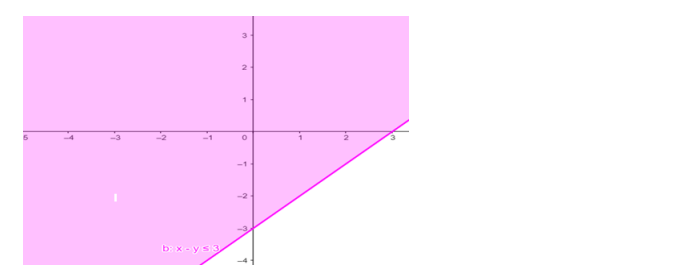# Solve the given inequalityQuestion:

Solve the given inequality $x-y \leq 3$ graphically in two - dimensional plane.

Solution:

The graphical representation of $x-y \leq 3$ is given by blue line in the figure below.

This lines divides $x-y$ plane into two parts.

Select a point (not on the line), which lies on one of the two parts, to determine whether the point satisfies the given inequality or not.

We select the point as $(0,0)$

It is observed that $0-0 \leq 3$ or $0 \leq 3$ which is true.

Therefore, the solution for the given inequality including the points on the line.

This can be represented as follows,